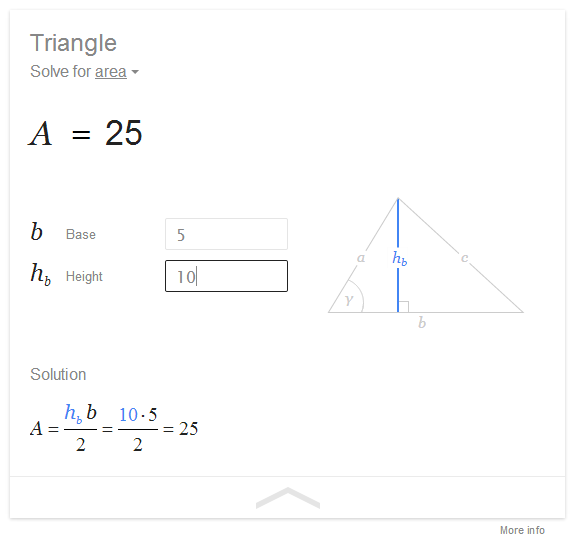An unofficial blog that watches Google's attempts to move your operating system online since 2005. Not affiliated with Google.

## March 19, 2014

Google has an interactive search widget that solves geometry problems. You can search for [solve triangle], [area of a circle], [volume of a sphere], [perimeter of a trapezoid], [area of a pentagon], [hypotenuse calculator], [triangular prism volume], [calculate the diagonal of a cube with 6mm edge], [volume of a cylinder with radius 4cm and height 8cm], [a^2+b^2=c^2 calc a=4 b=7 c=?] and more.Click the arrow and Google will show you the solution. Click one of the input boxes and the value is highlighted."Supported shapes: 2 and 3 dimensional curved shapes, platonic solids, polygons, prisms, pyramids, quadrilaterals, and triangles. Supported formulas and equations: Area, circumference, law of sines and cosines, hypotenuse, perimeter, Pythagorean theorem, surface area, and volume."

{ Thanks, Jonah Langlieb. }